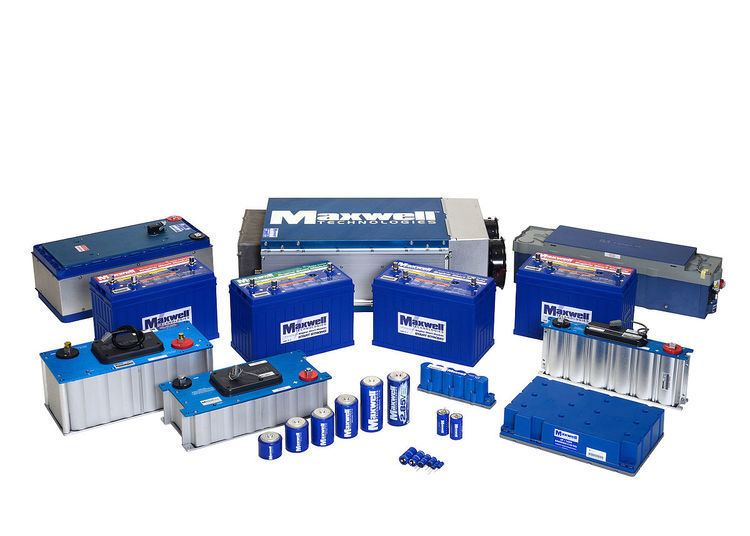# Electric double layer capacitor

Updated on
Covid-19Electric double-layer capacitors (EDLC) are electrochemical capacitors which energy storage predominant is achieved by Double-layer capacitance. In the past, all electrochemical capacitors were called "double-layer capacitors". However, since some years it is known that double-layer capacitors together with pseudocapacitors are part of a new family of electrochemical capacitors called supercapacitors, also known as ultracapacitors. Supercapacitors do not have a conventional solid dielectric.

The capacitance value of a supercapacitor is determined by two storage principles:

• Double-layer capacitance – electrostatic storage of the electrical energy achieved by separation of charge in a Helmholtz double layer at the interface between the surface of a conductor electrode and an electrolytic solution electrolyte. The separation of charge distance in a double-layer is on the order of a few Ångströms (0.3–0.8 nm) and is static in origin.
• Pseudocapacitance – Electrochemical storage of the electrical energy, achieved by redox reactions electrosorption or intercalation on the surface of the electrode by specifically adsorbed ions that results in a reversible faradaic charge-transfer on the electrode.
• Double-layer capacitance and pseudocapacitance both contribute inseparable to the total capacitance value of a supercapacitor. However, the ratio of the two can vary greatly, depending on the design of the electrodes and the composition of the electrolyte. Pseudocapacitance can increase the capacitance value by as much as an order of magnitude over that of the double-layer by itself.

Supercapacitors are divided into three family members, based on the design of the electrodes:

• Double-layer capacitors – with carbon electrodes or derivatives with much higher static double-layer capacitance than the faradaic pseudocapacitance
• Pseudocapacitors – with electrodes made of metal oxides or conducting polymers with much higher faradaic pseudocapacitance than the static double-layer capacitance
• Hybrid capacitors – capacitors with special electrodes that exhibit both significant double-layer capacitance and pseudocapacitance, such as lithium-ion capacitors
• However, because double-layer capacitance and pseudocapacitance both contribute inseparable to the total capacitance value of an electrochemical capacitor, a correct description of these capacitors only can be given under the generic term, see Supercapacitor.

## Commercial double layer capacitors

More specifically, commercial EDLCs in which energy storage predominant is achieved by double-layer capacitance, energy is stored by forming an electrical double layer of electrolyte ions on the surface of conductive electrodes. Since EDLCs are not limited by the electrochemical charge transfer kinetics of batteries, it can charge and discharge at a lot higher rate with lifetimes of more than 1 million cycles. The EDLC energy density is determined by operating voltage and the specific capacitance (farad/gram or farad/cm3) of the electrode/electrolyte system. The specific capacitance is related to the Specific Surface Area (SSA) accessible by the electrolyte, its interfacial double-layer capacitance, and the electrode material density.

Commercial EDLCs are based on two symmetric electrodes impregnated with electrolytes comprising tetraethylammonium tetrafluoroborate salts in organic solvents. Current EDLC with organic electrolytes operates at 2.7 V, reach energy densities around 5-8 Wh/kg and 7 to 10 Wh/l. The specific capacitance is related to the Specific Surface Area (SSA) accessible by the electrolyte, its interfacial double-layer capacitance, and the electrode material density. Graphene-based platelets with mesoporous spacer material is a promising structure for increasing the SSA of the electrolyte.

Topics